Courses

# Electromagnetic Induction MCQ Level - 1

## 10 Questions MCQ Test Topic wise Tests for IIT JAM Physics | Electromagnetic Induction MCQ Level - 1

Description
This mock test of Electromagnetic Induction MCQ Level - 1 for IIT JAM helps you for every IIT JAM entrance exam. This contains 10 Multiple Choice Questions for IIT JAM Electromagnetic Induction MCQ Level - 1 (mcq) to study with solutions a complete question bank. The solved questions answers in this Electromagnetic Induction MCQ Level - 1 quiz give you a good mix of easy questions and tough questions. IIT JAM students definitely take this Electromagnetic Induction MCQ Level - 1 exercise for a better result in the exam. You can find other Electromagnetic Induction MCQ Level - 1 extra questions, long questions & short questions for IIT JAM on EduRev as well by searching above.
QUESTION: 1

### A bar magnetic is dropped between a current carrying coil. What would be its acceleration?

Solution:

When a magnet is dropped between current carrying coil then magnetic flux in coil changes due to which induce current produces in the coil, the direction of induce current will be such that it oppose the motion of magnet, so acceleration of magnet will be less than g

The correct answer is: less than g downwards

QUESTION: 2

### Lenz’s law gives

Solution:

According to Lenz’s law” the direction induced current is always in such a direction that it opposes the cause due to which it is produced

The correct answer is: the direction of the induced current

QUESTION: 3

### The self inductance of a solenoid of length L, area of cross section A and having N turns is :

Solution:

The correct answer is: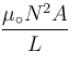QUESTION: 4

A copper ring is tied to a string and suspended vertically. On bringing a magnetic towards the coil, as shown in the figure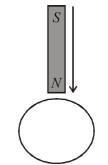Solution:

The correct answer is: the ring will move away from magnet

QUESTION: 5

A metallic rod completes its circuit as shown in the figure. The circuit is normal to a magnetic field  B = 0.15 tesla. If the resistances of the rod is 3Ω the force required to move the rod with a constant velocity of 2m/sec is :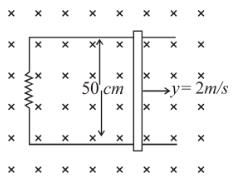Solution: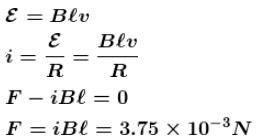The correct answer is: 3.75 × 10–3 N

QUESTION: 6

The plate circular coils P and Q have radii r1 and  r2. (r1 << r2) and are coaxial as shown in figure. the number of turns in P and Q are respectively N1 and  N2. If current in coil Q is varied steadily at a rate x A/s then the induced emf in the coil P will be approximately :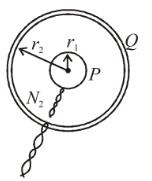Solution: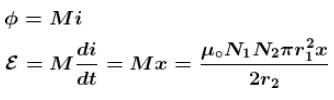The correct answer is: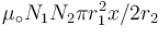QUESTION: 7

A metal rod of length L is placed normal to a magnetic field and rotated through one end of rod in a circular path with frequency f. The potential difference between it ends will be :

Solution: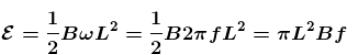QUESTION: 8

A circular conducting loop is placed in uniform magnetic field B = 0.025 Tesla  perpendicular to plane of loop. The loop is allowed to shrink in radius at rate mm/second. When radius is cm. What is the value of instantaneous induced EMF.

Solution: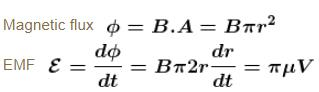QUESTION: 9

The graph between the current and the time for an inductance coil is shown below which of the following graph show the voltage time variation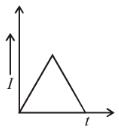Solution: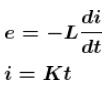The correct answer is: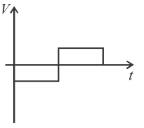QUESTION: 10

In a step down transformer input voltage is 200 volt and output voltage 5 volt. The ratio of number of turns in it will be

Solution: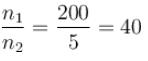The correct answer is: 40 : 1